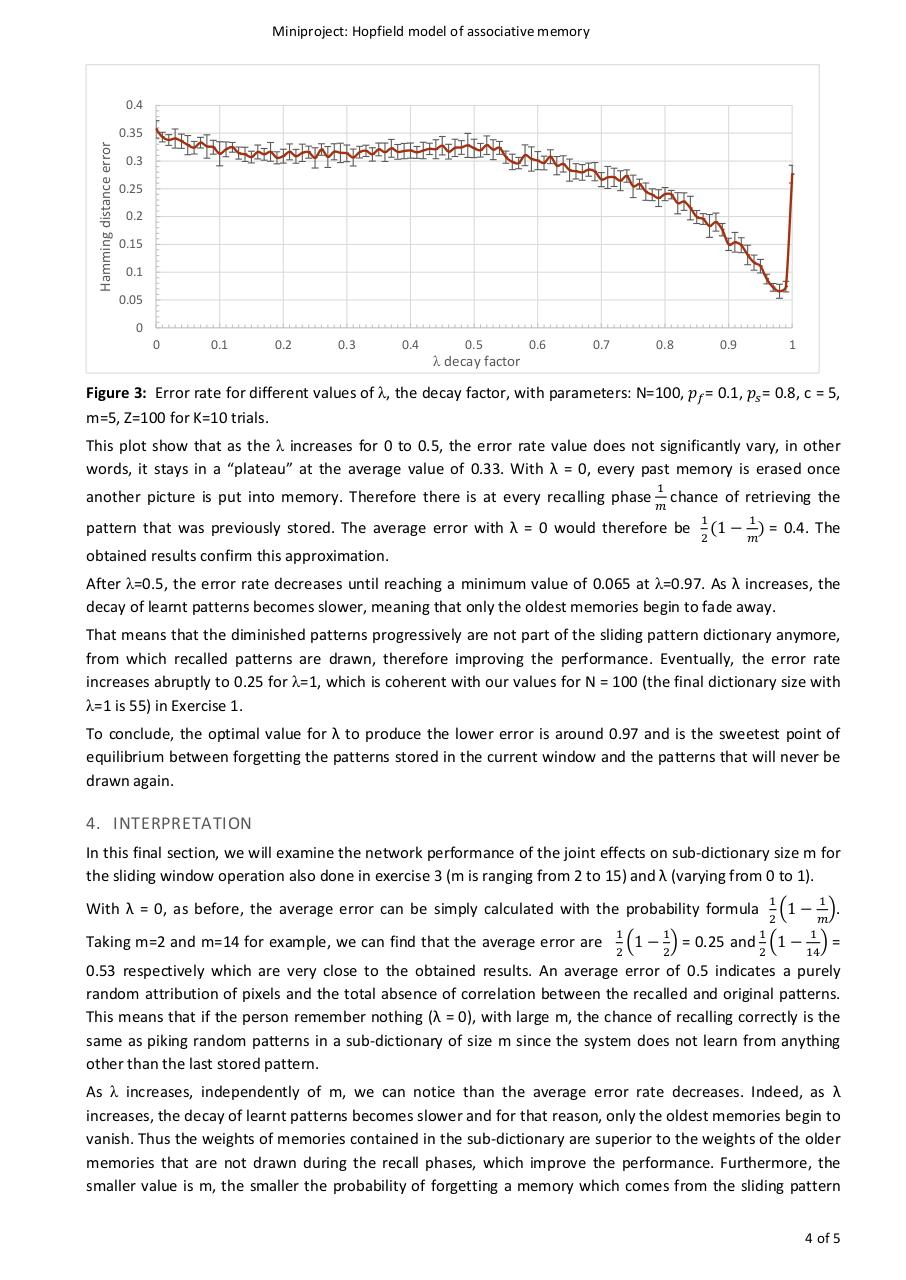Projet Hopfield.pdfPage 1 2 3 4 5 6

Text preview

Miniproject: Hopfield model of associative memory

0.4

Hamming distance error

0.35
0.3
0.25
0.2
0.15
0.1
0.05
0
0

0.1

0.2

0.3

0.4

0.5

λ decay factor

0.6

0.7

0.8

0.9

1

Figure 3: Error rate for different values of λ, the decay factor, with parameters: N=100, 𝑝𝑝𝑓𝑓 = 0.1, 𝑝𝑝𝑠𝑠 = 0.8, c = 5,
m=5, Z=100 for K=10 trials.

This plot show that as the λ increases for 0 to 0.5, the error rate value does not significantly vary, in other
words, it stays in a “plateau” at the average value of 0.33. With λ = 0, every past memory is erased once
another picture is put into memory. Therefore there is at every recalling phase

1
𝑚𝑚

chance of retrieving the

pattern that was previously stored. The average error with λ = 0 would therefore be
obtained results confirm this approximation.

1
1
(1 − ) =
2
𝑚𝑚

0.4. The

After λ=0.5, the error rate decreases until reaching a minimum value of 0.065 at λ=0.97. As λ increases, the
decay of learnt patterns becomes slower, meaning that only the oldest memories begin to fade away.
That means that the diminished patterns progressively are not part of the sliding pattern dictionary anymore,
from which recalled patterns are drawn, therefore improving the performance. Eventually, the error rate
increases abruptly to 0.25 for λ=1, which is coherent with our values for N = 100 (the final dictionary size with
λ=1 is 55) in Exercise 1.
To conclude, the optimal value for λ to produce the lower error is around 0.97 and is the sweetest point of
equilibrium between forgetting the patterns stored in the current window and the patterns that will never be
drawn again.

4. INTERPRETATION
In this final section, we will examine the network performance of the joint effects on sub-dictionary size m for
the sliding window operation also done in exercise 3 (m is ranging from 2 to 15) and λ (varying from 0 to 1).
1
1
�1 − �.
2
𝑚𝑚
1
1
and �1 − � =
2
14

With λ = 0, as before, the average error can be simply calculated with the probability formula
Taking m=2 and m=14 for example, we can find that the average error are

1
1
�1 − � =
2
2

0.25

0.53 respectively which are very close to the obtained results. An average error of 0.5 indicates a purely
random attribution of pixels and the total absence of correlation between the recalled and original patterns.
This means that if the person remember nothing (λ = 0), with large m, the chance of recalling correctly is the
same as piking random patterns in a sub-dictionary of size m since the system does not learn from anything
other than the last stored pattern.

As λ increases, independently of m, we can notice than the average error rate decreases. Indeed, as λ
increases, the decay of learnt patterns becomes slower and for that reason, only the oldest memories begin to
vanish. Thus the weights of memories contained in the sub-dictionary are superior to the weights of the older
memories that are not drawn during the recall phases, which improve the performance. Furthermore, the
smaller value is m, the smaller the probability of forgetting a memory which comes from the sliding pattern
4 of 5